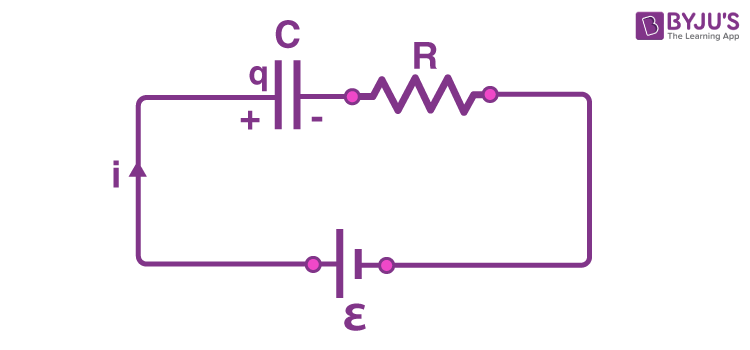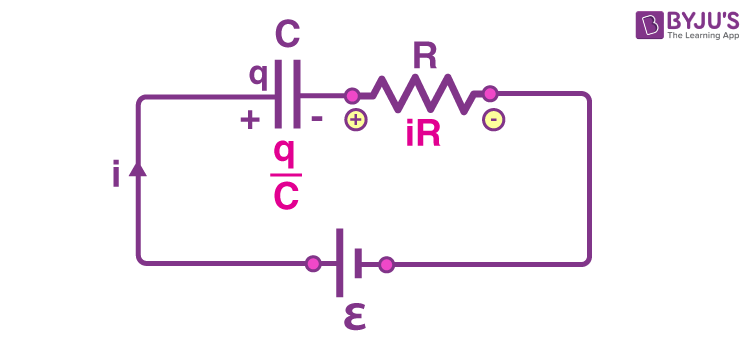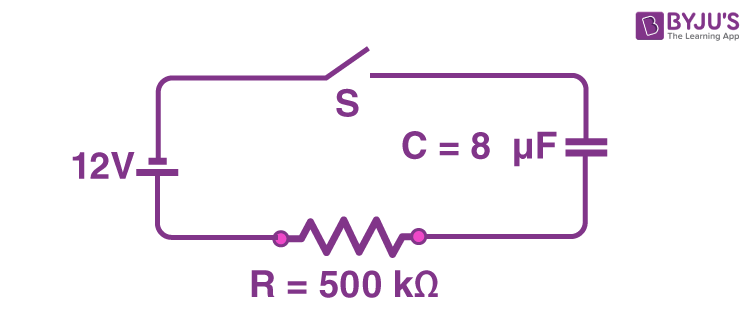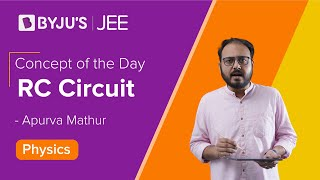Win up to 100% scholarship on Aakash BYJU'S JEE/NEET courses with ABNAT Win up to 100% scholarship on Aakash BYJU'S JEE/NEET courses with ABNAT

# RC Circuit

A resistor-capacitor circuit (RC CIrcuit) is an electrical circuit consisting of passive components like resistors and capacitors, driven by the current source or the voltage source.

The capacitor stores energy and the resistor connected to the circuit control the rate of charging or discharging.

The charging and the discharging of the capacitor is not an instant process but takes some time. If the resistor and capacitor are connected in series, the capacitor charges gradually through the resistor, until the voltage across the resistor is equal to the supply voltage.

## Charging of CapacitorLet us consider that a fully discharged capacitor is connected to the circuit and the switch is open. The initial condition of the circuit is time (t) = 0, charge (q) = 0 and current (i) = 0. When the switch is closed, the time will start from t = 0, and the current starts flowing through the capacitor and the resistor. An initially uncharged capacitor can be assumed to be a connecting wire just after the circuit is completed.

At time t = 0, the potential difference across the capacitor is zero and continues to be equal to zero just after the time t = 0.

The current flowing at this time is called the charging current and it is calculated using Ohm’s law. The value of current is

i = i0 = ε/R.

Here, ε is the emf of the cell.

R is the resistance of the resistor.As time progresses, the capacitor charges up and a potential difference develops across the capacitor. Let us consider at any time “t”, the charge across the capacitor is “q” and the current in the circuit is “i”. The potential difference across the capacitor is q/C and the potential difference across the resistor is iR.

Applying Kirchoff’s voltage law, we get

-q/c -iR + ε = 0

(or) iR = ε – q/C

⇒ i = (cε – q)/RC = dq/dt [ since i = dq/dt]

$$\begin{array}{l}\Rightarrow \frac{dq}{C\varepsilon -q}=\frac{1}{RC}dt\end{array}$$

Integrating on both sides,

$$\begin{array}{l}\int_{0}^{q}\frac{dq}{C\varepsilon -q}=\int_{0}^{t}\frac{1}{RC}dt\end{array}$$
$$\begin{array}{l}\left [ \frac{ln(C\varepsilon -q) }{-1}\right ]_{0}^{q}= \frac{1}{RC}[t]_{0}^{t}\end{array}$$
$$\begin{array}{l}\left [ \frac{ln(C\varepsilon -q) }{C\varepsilon }\right ]= \frac{-t}{RC}\end{array}$$
$$\begin{array}{l}\frac{(C\varepsilon -q) }{C\varepsilon }= e^{\frac{-t}{RC}}\end{array}$$

⇒ q = CƐ (1- e-t/RC)

Differentiating the above equation we get the value of current

$$\begin{array}{l}i = \frac{dq}{dt}= \frac{\varepsilon }{R}e^{\frac{-t}{RC}}\end{array}$$

The current is called transient current and it depends on time.

## Time Constant

The time constant (τ) during the charging of the capacitor is the time required to increase the charge on the capacitor by 37% of its final charge. It is the measure of how fast the capacitor can be charged.

time constant (τ) = RC

### Solved Examples

Question 1: A capacitor of capacitance 1000 μF is connected to a resistor of resistance 150 kΩ and a battery of 1.5 V in series. Find the maximum current that flows through the resistor while charging.

I = Ɛ/R = 1.5 V/150 kΩ = 0.01 mA

Question 2: A 50 V AC is applied across an RC series network. The RMS voltage across the resistance is 40 V, then the potential across the capacitance would be

a) 10 V

b) 20 V

c) 30 V

d) 40 V

Solution:

For the RC circuit,

$$\begin{array}{l}V = \sqrt{V_{R}^{2}+V_{C}^{2}}\end{array}$$
$$\begin{array}{l}50 = \sqrt{40^{2}+V_{C}^{2}}\end{array}$$
$$\begin{array}{l}\Rightarrow V_{c}= 30 V\end{array}$$

Question 3: The impedance of the RC circuit is Z1 for the frequency f, and Z2 for frequency 2f. Then, Z1/Z2 is

a) between 1 and 2

b) 2

c) Between ½ and 1

d) ½

Answer: a) between 1 and 2

Solution:

For the frequency f1,

$$\begin{array}{l}Z_{1}= \sqrt{R^{2}+\frac{1}{4\pi ^{2}f^{2}C^{2}}}\end{array}$$

For the frequency f2,

$$\begin{array}{l}Z_{1}= \sqrt{R^{2}+\frac{1}{4\pi ^{2}2f^{2}C^{2}}}= \sqrt{R^{2}+\frac{1}{16\pi ^{2}f^{2}C^{2}}}\end{array}$$
$$\begin{array}{l}\frac{Z_{1}^{2}}{Z_{2}^{2}}= \frac{{R^{2}+\frac{1}{4\pi ^{2}2f^{2}C^{2}}}}{{R^{2}+\frac{1}{16\pi ^{2}f^{2}C^{2}}}}\end{array}$$

Z1/Z2 lies between 1 and 2.

Question 4: An uncharged capacitor and a resistor are connected in series as shown in the figure below. The emf of the battery is ε = 12 V, C = 8 μF , R = 500 kΩ

After the switch is closed, find

(a) The time constant of the RC circuit.

(b) The maximum charge on the capacitor.

(c) The charge on the capacitor 6 s after the switch is closed.Solution:

(a) The time constant of the RC circuit, τ = (500 x 10-3 Ω) (8 x 10-6) = 4S

(b) Q = Qf (1 – e-t/RC)

Qf = CƐ is the final charge

The maximum charge on the capacitor, Qmax = CƐ

= (8 x 10-6) (12) = 96 μC

(c) The charge on the capacitor 6 s after the switch is closed is given by

Q = Qf (1 – e-t/τ)

= 96 (1 – e -6/4)

= 74.5 μC

## Video Lesson – RC circuit## Frequently Asked Questions on the RC circuit

### What does an RC series circuit necessarily imply?

A series RC circuit is made up of a resistor and a capacitor linked in series to an AC source.

### Where do you see RC circuits in everyday life?

In a circuit, capacitors and resistors are frequently seen together. In real life, such RC circuits are common. They are employed in camera flashes, heart pacemakers, to control the speed of a car’s windshield wipers, the timing of traffic signals and a variety of other electrical equipment.

### How does an RC circuit work?

The capacitor stores energy and the rate of charging or discharging is controlled by the resistor attached to the circuit.